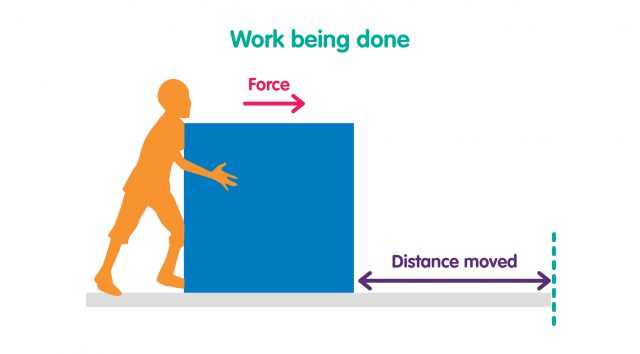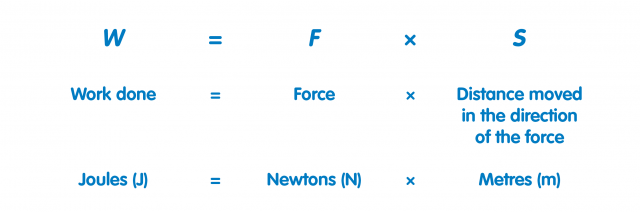# Physics - Work done and power

?
• Created by: Dan_23
• Created on: 26-05-21 20:23
1. Work is done when a force moves an object through a distance

Work is done when a force moves an object through a distance.

For example, if a person pushes a box along the floor, they are doing work on the box.The object must move in the same direction as the force.

Work done can be calculated from the force and the distance moved in the direction of the force.Work done is the same as energy transferred.

The units of work done are joules.

1 joule is the same as 1 newton-metre.

The greater the force acting on an object, the greater the work done or energy transferred.

The greater the distance moved by the object, the greater the work done or energy transferred.

1 joule of work is done when a force of 1 N causes a displacement of 1 m.

1. Work done against friction causes a temperature rise